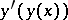# Differential-functional equation

(diff) ← Older revision | Latest revision (diff) | Newer revision → (diff)
An equation relating the argument and the unknown function and its derivatives, generally taken with a functionally transformed argument. Here, the expression of the functional transformation may include the unknown function, as a result of which the equation may contain combinations such as, etc. The concept of a differential-functional equation is often understood to be the synonym of an ordinary differential equation with deviating or distributed arguments (cf. Differential equations, ordinary, with distributed arguments).